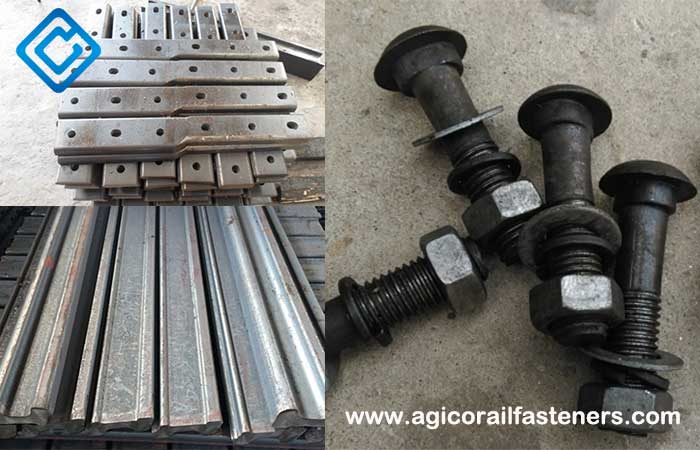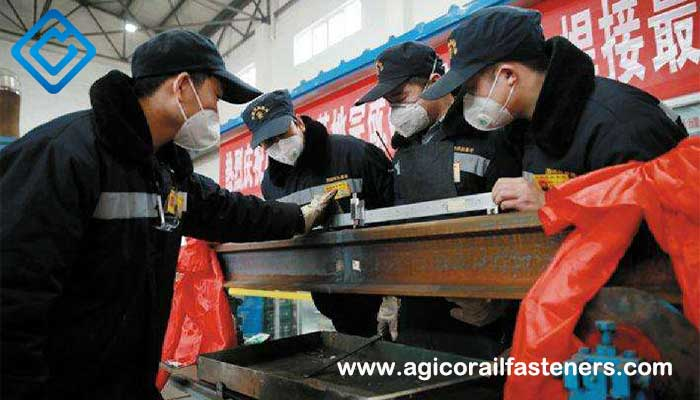# How To Replace The Damaged Railroad Steel Rails?

Frequently, there are always damaged railroad steel rails along the railways, so how to replace the damaged railroad steel rails? Rail replacement includes two types: the replacement of continuous welded rail (CWR) and the replacement of welded railroad steel rail. The CWR railway refers to weld two ends of short rails together, which will form a few kilometers length of long railroad steel rail with the advantage of the elimination of the rail joints and disadvantage of being easily impact by temperature changes.For the welded rail, the replacement is only needed to cut old damaged railroad steel rails section and install another new one by fixing fish bolts and nuts. For underground railways like metro, the ambient temperature is basically constant and unchangeable. So just replacing the damaged with new ones directly and welding both ends is ok. For open-air CWR railways, it is more difficult because we have to consider the temperature changes. The thermal expansion and contraction caused by temperature changes will greatly impact the railroad steel rail replacement. So here we must know the concept of stress-free temperature that refers to the rail temperature after the welding completion with relevant fasteners.

That is to say, as we cut the rail open, if the temperature is equal to stress-free temperature, everything is ok; if the temperature is lower than stress-free temperature, the railroad steel rails will retract a certain length. This is resulted out of the temperature difference.## Determination of Temperature Force

The rail expansion and contraction force is only related to the temperature force Pt, and Pt = Pt =αEF*Δt =2.5FΔt, F stands for the fixed value for rail sectional area, for example, for 60kg/m rail, F=7745mm. For Pt =19363Δt, as the temperature change is a fixed value, the temperature force of the railroad steel rail is also a fixed value, commonly used temperature is as shown in the following table:

Δt(℃) Pt(N)
3 58089
5 96815
10 193630
15 290445

L= 625P t/R (mm) R: resistance value of each fastener, 625mm stands for the spacing of adjacent two fasteners, and Pt /R needs an integer.

R=2*r*f
Here, r stands for the buckling pressure value of each fastener, the minimum design value of each small resistance fasteners is 5600N; f stands for friction coefficient, F value is 0.39, the most unfavorable value is 0.2, Obtain：
L= 312.5Pt /rf (mm)
L=6050937.5t /rf (mm)

### Theoretical value of resistance:

As r=5600N, f=0.39, theoretical value of fastener resistance:
L=0.143Pt =2770.6Δt (mm)

### The most unfavorable situation of fastener resistance:

As r=5600N*70%=3920N, f=0.2, the most unfavorable value is:
L=0.399 Pt=7718.0Δt(mm)

## Calculation of Expansion and Contraction

Δl=α*L*Δt
α stands for the coefficient of rail expansion, it is 0.0000118/℃
L stands for length of rail expansion zone,
Δl stands for expansion and contraction value
(1) Theoretical value of expansion and contraction of fastener is :
As r=5600N, f=0.39, theoretical value of expansion and contraction of fastener is L=0.0327Δt2 (mm)
(2) The most unfavorable situation of fastener expansion and contraction of fastener is:
As r=5600N*70%=3920N, f=0.2,the most unfavorable value is: L=0.0911Δt (mm)

Note: Due to the fracture of the railroad steel rail from the middle, the caused both ends will have the thermal expansion and contraction, so Δl is a single end value, the total value of 2Δl.

## Calculation of Joint Resistance

Bolt tension: P=T/ (K*D)
K stands for torque coefficient, and the value range is 0.18-0.23;
D stands for bolt screw diameter (mm): 26mm;
T stands for joint bolt torque: 1200N*M
Obtain:
P=T/ (K*D) =1200/ (0.2*26) =230.8KN
Total joint resistance: PH=6f*P*cosφ/sin(α+φ)
Here f stands for friction coefficient, the new fastener for 0.5-0.6, old fastener for 0.18-0.2.The torque coefficient and joint resistance of joint bolt for 60kg/m railroad steel rails are shown in the following table:

Torque(N*m) Joint Resistance(KN)
800 490
900 510
1000 570

It is concluded from the table that the resistance of the joint is much higher than that of the temperature as Δt<20℃, so it is concluded that the joint bolt can bear the temperature force.

Get in touch